# sklearn_statmodels_linear_regression

## Regression with scikit-learn and statmodels¶¶

This notebook demonstrates how to conduct a valid regression analysis using a combination of Sklearn and statmodels libraries. While sklearn is popular and powerful from an operational point of view, it does not provide the detailed metrics required to statistically analyze your model, evaluate the importance of predictors, build or simplify your model.

We use other libraries like statmodels or scipy.stats to bridge this gap.

ToC

### Scikit-learn¶¶

Scikit-learn is one of the science kits for SciPy stack. Scikit has a collection of prediction and learning algorithms, grouped into

• classification
• clustering
• regression
• dimensionality reduction

Each algorithm follows a typical pattern with a fit, predict method. In addition you get a set of utility methods that help with splitting datasets into train-test sets and for validating the outputs.

In :
import pandas as pd
import matplotlib.pyplot as plt
%matplotlib inline
import seaborn as sns


### Predicting housing prices without data normalization¶¶

#### Exploratory data anslysis (EDA)¶¶

In :
usa_house = pd.read_csv('../udemy_ml_bootcamp/Machine Learning Sections/Linear-Regression/USA_housing.csv')

Out:
Avg Income Avg House Age Avg. Number of Rooms Avg. Number of Bedrooms Area Population Price Address
0 79545.45857 5.682861 7.009188 4.09 23086.80050 1.059034e+06 208 Michael Ferry Apt. 674\nLaurabury, NE 3701…
1 79248.64245 6.002900 6.730821 3.09 40173.07217 1.505891e+06 188 Johnson Views Suite 079\nLake Kathleen, CA…
2 61287.06718 5.865890 8.512727 5.13 36882.15940 1.058988e+06 9127 Elizabeth Stravenue\nDanieltown, WI 06482…
3 63345.24005 7.188236 5.586729 3.26 34310.24283 1.260617e+06 USS Barnett\nFPO AP 44820
4 59982.19723 5.040555 7.839388 4.23 26354.10947 6.309435e+05 USNS Raymond\nFPO AE 09386
In :
usa_house.info()

<class 'pandas.core.frame.DataFrame'>
RangeIndex: 5000 entries, 0 to 4999
Data columns (total 7 columns):
Avg Income                 5000 non-null float64
Avg House Age              5000 non-null float64
Avg. Number of Rooms       5000 non-null float64
Avg. Number of Bedrooms    5000 non-null float64
Area Population            5000 non-null float64
Price                      5000 non-null float64
dtypes: float64(6), object(1)
memory usage: 273.5+ KB

In :
usa_house.describe()

Out:
Avg Income Avg House Age Avg. Number of Rooms Avg. Number of Bedrooms Area Population Price
count 5000.000000 5000.000000 5000.000000 5000.000000 5000.000000 5.000000e+03
mean 68583.108984 5.977222 6.987792 3.981330 36163.516039 1.232073e+06
std 10657.991214 0.991456 1.005833 1.234137 9925.650114 3.531176e+05
min 17796.631190 2.644304 3.236194 2.000000 172.610686 1.593866e+04
25% 61480.562390 5.322283 6.299250 3.140000 29403.928700 9.975771e+05
50% 68804.286405 5.970429 7.002902 4.050000 36199.406690 1.232669e+06
75% 75783.338665 6.650808 7.665871 4.490000 42861.290770 1.471210e+06
max 107701.748400 9.519088 10.759588 6.500000 69621.713380 2.469066e+06

Find the correlation between each of the numerical columns to the house price

In :
sns.pairplot(usa_house)

Out:
<seaborn.axisgrid.PairGrid at 0x108258ef0>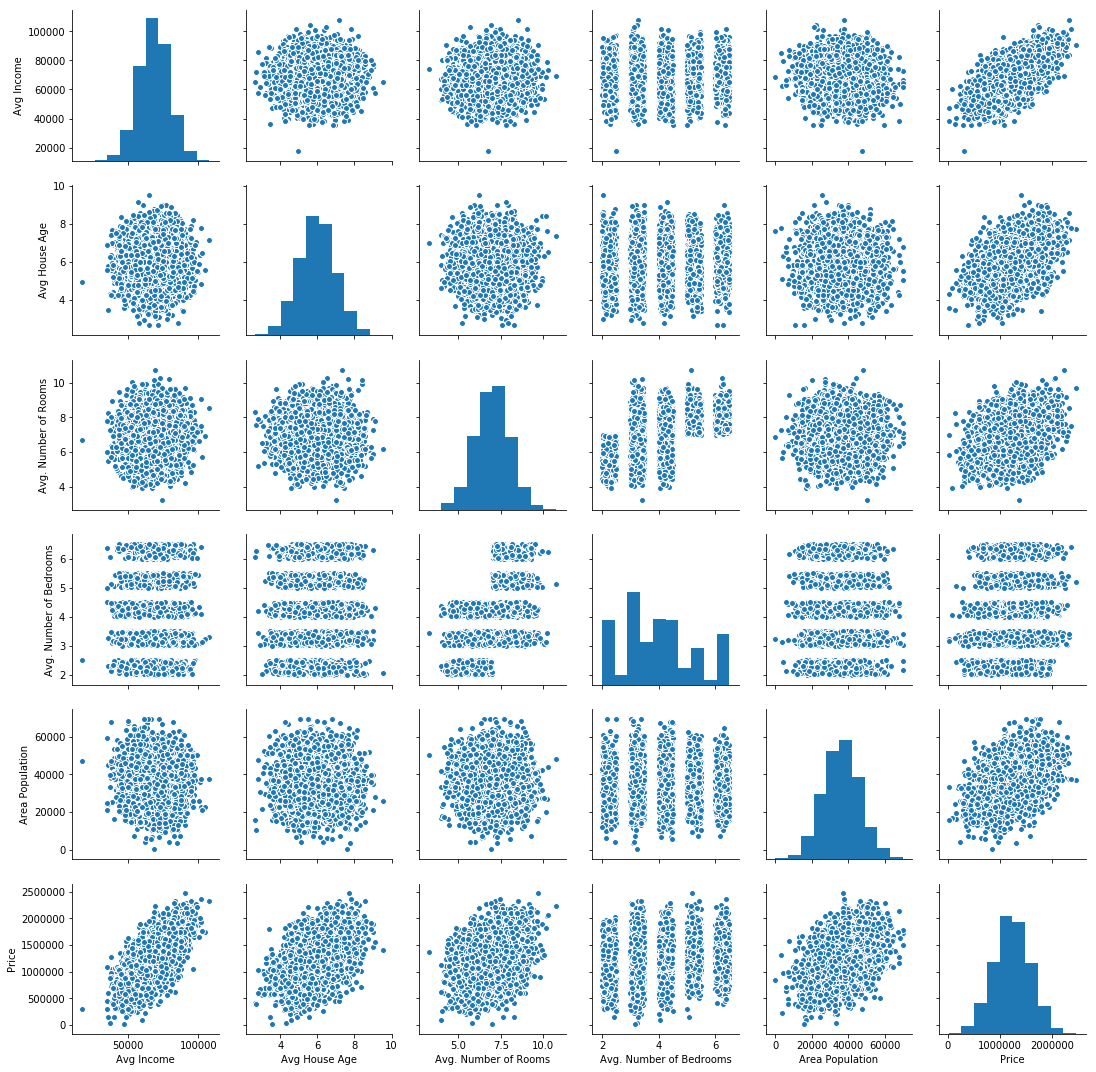From this chart, we now,

• distribution of house price is normal (last chart)
• some scatters show a higher correlation, while some other show no correlation.
In :
fig, axs = plt.subplots(1,2, figsize=[15,5])
sns.distplot(usa_house['Price'], ax=axs)
sns.heatmap(usa_house.corr(), ax=axs, annot=True)
fig.tight_layout()

/Users/atma6951/anaconda3/envs/pychakras/lib/python3.6/site-packages/matplotlib/axes/_axes.py:6462: UserWarning: The 'normed' kwarg is deprecated, and has been replaced by the 'density' kwarg.
warnings.warn("The 'normed' kwarg is deprecated, and has been "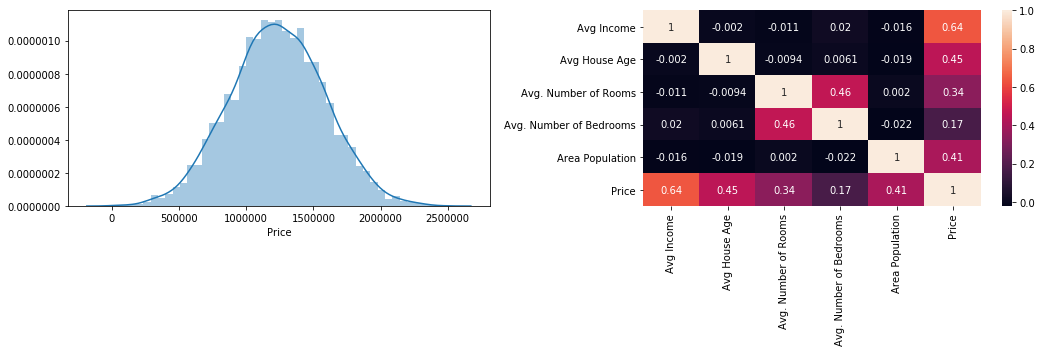#### Data cleaning¶¶

Throw out the text column and split the data into predictor and predicted variables

In :
usa_house.columns

Out:
Index(['Avg Income', 'Avg House Age', 'Avg. Number of Rooms',
'Avg. Number of Bedrooms', 'Area Population', 'Price', 'Address'],
dtype='object')
In :
X = usa_house[['Avg Income', 'Avg House Age', 'Avg. Number of Rooms',
'Avg. Number of Bedrooms', 'Area Population']]

y = usa_house[['Price']]

##### Train test split¶¶
In :
from sklearn.model_selection import train_test_split
X_train, X_test, y_train, y_test = train_test_split(X, y, test_size=0.33)

In :
len(X_train)

Out:
3350
In :
len(X_test)

Out:
1650
In :
X_test.head()

Out:
Avg Income Avg House Age Avg. Number of Rooms Avg. Number of Bedrooms Area Population
1066 64461.39215 7.949614 6.675121 2.04 34210.93608
4104 61687.39442 5.507913 6.995603 3.34 45279.16397
662 69333.68219 5.924392 6.542682 2.00 17187.11819
2960 74095.71281 5.908765 6.847362 3.00 32774.02197
1604 53066.37227 6.754571 8.062652 3.23 19103.12711

#### Multiple regression¶¶

We use a number of numerical columns to regress the house price. Each column’s influence will vary, just like in real life, the number of bedrooms might not influence as much as population density. We can determine the influence from the correlation shown in the heatmap above

In :
from sklearn.linear_model import LinearRegression
lm = LinearRegression()

##### Fit¶¶
In :
lm.fit(X_train, y_train) #no need to capture the return. All is stored in lm

Out:
LinearRegression(copy_X=True, fit_intercept=True, n_jobs=1, normalize=False)

Create a table showing the coefficient (influence) of each of the columns

In :
cdf = pd.DataFrame(lm.coef_, index=X_train.columns, columns=['coefficients'])
cdf

Out:
coefficients
Avg Income 21.639515
Avg House Age 166094.788683
Avg. Number of Rooms 119855.858430
Avg. Number of Bedrooms 3037.782793
Area Population 15.215241

Note, the coefficients for house age, number of rooms is pretty large. However that does not really mean they are more influential compared to income. It is simply because our dataset has not been normalized and the data range for each of these columns vary widely.

##### Predict¶¶
In :
y_predicted = lm.predict(X_test)
len(y_predicted)

Out:
1650

#### Accuracy assessment / Model validation¶¶

In :
plt.scatter(y_test, y_predicted) #actual vs predicted

Out:
<matplotlib.collections.PathCollection at 0x1a1b4f4668>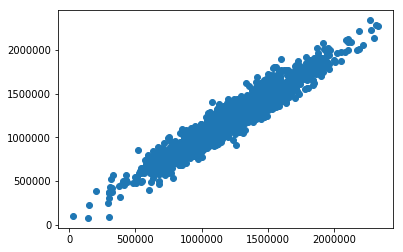##### Distribution of residuals¶¶
In :
sns.distplot((y_test - y_predicted))

/Users/atma6951/anaconda3/envs/pychakras/lib/python3.6/site-packages/matplotlib/axes/_axes.py:6462: UserWarning: The 'normed' kwarg is deprecated, and has been replaced by the 'density' kwarg.
warnings.warn("The 'normed' kwarg is deprecated, and has been "

Out:
<matplotlib.axes._subplots.AxesSubplot at 0x1a1b519c88>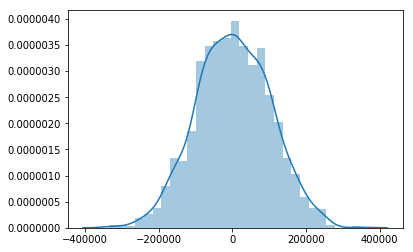##### Quantifying errors¶¶
In :
from sklearn import metrics
metrics.mean_absolute_error(y_test, y_predicted)

Out:
80502.80373530561

RMSE

In :
import numpy
numpy.sqrt(metrics.mean_squared_error(y_test, y_predicted))

Out:
99779.47354600814

#### Combine the predicted values with input¶¶

In :
X_test['predicted_price'] = y_predicted

/Users/atma6951/anaconda3/envs/pychakras/lib/python3.6/site-packages/ipykernel_launcher.py:1: SettingWithCopyWarning:
A value is trying to be set on a copy of a slice from a DataFrame.
Try using .loc[row_indexer,col_indexer] = value instead

See the caveats in the documentation: http://pandas.pydata.org/pandas-docs/stable/indexing.html#indexing-view-versus-copy
"""Entry point for launching an IPython kernel.

In :
X_test.head()

Out:
Avg Income Avg House Age Avg. Number of Rooms Avg. Number of Bedrooms Area Population predicted_price
1066 64461.39215 7.949614 6.675121 2.04 34210.93608 1.396405e+06
4104 61687.39442 5.507913 6.995603 3.34 45279.16397 1.141590e+06
662 69333.68219 5.924392 6.542682 2.00 17187.11819 8.904433e+05
2960 74095.71281 5.908765 6.847362 3.00 32774.02197 1.267610e+06
1604 53066.37227 6.754571 8.062652 3.23 19103.12711 8.913813e+05

### Predicting housing prices with data normalization and statmodels¶¶

As seen earlier, even though sklearn will perform regression, it is hard to compare which of the predictor variables are influential in determining the house price. To answer this better, let us standardize our data to Std. Normal distribution using sklearn preprocessing.

#### Scale the housing data to Std. Normal distribution¶¶

We use StandardScaler from sklearn.preprocessing to normalize each predictor to mean 0 and unit variance. What we end up with is z-score for each record.

$$z-score = \frac{x_{i} - \mu}{\sigma}$$
In :
from sklearn.preprocessing import StandardScaler
s_scaler = StandardScaler()

In :
# get all columns except 'Address' which is non numeric
usa_house.columns[:-1]

Out:
Index(['Avg Income', 'Avg House Age', 'Avg. Number of Rooms',
'Avg. Number of Bedrooms', 'Area Population', 'Price'],
dtype='object')
In :
usa_house_scaled = s_scaler.fit_transform(usa_house[usa_house.columns[:-1]])
usa_house_scaled = pd.DataFrame(usa_house_scaled, columns=usa_house.columns[:-1])

Out:
Avg Income Avg House Age Avg. Number of Rooms Avg. Number of Bedrooms Area Population Price
0 1.028660 -0.296927 0.021274 0.088062 -1.317599 -0.490081
1 1.000808 0.025902 -0.255506 -0.722301 0.403999 0.775508
2 -0.684629 -0.112303 1.516243 0.930840 0.072410 -0.490211
3 -0.491499 1.221572 -1.393077 -0.584540 -0.186734 0.080843
4 -0.807073 -0.944834 0.846742 0.201513 -0.988387 -1.702518
In :
usa_house_scaled.describe().round(3) # round the numbers for dispaly

Out:
Avg Income Avg House Age Avg. Number of Rooms Avg. Number of Bedrooms Area Population Price
count 5000.000 5000.000 5000.000 5000.000 5000.000 5000.000
mean 0.000 -0.000 -0.000 -0.000 0.000 0.000
std 1.000 1.000 1.000 1.000 1.000 1.000
min -4.766 -3.362 -3.730 -1.606 -3.626 -3.444
25% -0.666 -0.661 -0.685 -0.682 -0.681 -0.664
50% 0.021 -0.007 0.015 0.056 0.004 0.002
75% 0.676 0.679 0.674 0.412 0.675 0.677
max 3.671 3.573 3.750 2.041 3.371 3.503

#### Train test split¶¶

In :
X_scaled = usa_house_scaled[['Avg Income', 'Avg House Age', 'Avg. Number of Rooms',
'Avg. Number of Bedrooms', 'Area Population']]

y_scaled = usa_house_scaled[['Price']]

In :
Xscaled_train, Xscaled_test, yscaled_train, yscaled_test = \
train_test_split(X_scaled, y_scaled, test_size=0.33)


#### Train the model¶¶

In :
lm_scaled = LinearRegression()
lm_scaled.fit(Xscaled_train, yscaled_train)

Out:
LinearRegression(copy_X=True, fit_intercept=True, n_jobs=1, normalize=False)
In :
cdf_scaled = pd.DataFrame(lm_scaled.coef_,
index=Xscaled_train.columns, columns=['coefficients'])
cdf_scaled

Out:
coefficients
Avg Income 0.653151
Avg House Age 0.462883
Avg. Number of Rooms 0.341197
Avg. Number of Bedrooms 0.007156
Area Population 0.424653
In :
lm_scaled.intercept_

Out:
array([0.00215375])

From the table above, we notice Avg Income has more influence on the Price than other variables. This was not apparent before scaling the data. Further this corroborates the correlation matrix produced during exploratory data analysis.

#### Evaluate model parameters using statsmodels¶¶

statmodels is a different Python library built for and by statisticians. Thus it provides a lot more information on your model than sklearn. We use it here to refit against the data and evaluate the strength of fit.

In :
import statsmodels.api as sm
import statsmodels

In :
from statsmodels.regression import linear_model

In :
yscaled_train.shape

Out:
(3350, 1)
In :
Xscaled_train = sm.add_constant(Xscaled_train)
sm_ols = linear_model.OLS(yscaled_train, Xscaled_train) # i know, the param order is inverse
sm_model = sm_ols.fit()

In :
sm_model.summary()

Out:
Dep. Variable: R-squared: Price 0.918 OLS 0.917 Least Squares 7446. Thu, 23 Aug 2018 0.00 16:25:05 -552.85 3350 1118. 3344 1154. 5 nonrobust
coef std err t P>|t| [0.025 0.975] -0.0015 0.005 -0.311 0.756 -0.011 0.008 0.6532 0.005 129.977 0.000 0.643 0.663 0.4629 0.005 93.799 0.000 0.453 0.473 0.3412 0.006 61.197 0.000 0.330 0.352 0.0072 0.006 1.277 0.202 -0.004 0.018 0.4247 0.005 86.506 0.000 0.415 0.434
 Omnibus: Durbin-Watson: 11.426 1.995 0.003 9.047 0.023 0.0109 2.75 1.66

The regression coefficients are identical between sklearn and statsmodels libraries. The $R^{2}$ of 0.919 is as high as it gets. This indicates the predicted (train) Price varies similar to actual. Another measure of health is the S (std. error) and p-value of coefficients. The S of Avg. Number of Bedrooms is as low as other predictors, however it has a high p-value indicating a low confidence in predicting its coefficient.

Similar is the p-value of the intercept.

#### Predict for unkown values¶¶

In :
yscaled_predicted = lm_scaled.predict(Xscaled_test)
residuals_scaled = yscaled_test - yscaled_predicted


#### Evaluate model using charts¶¶

In addition to the numerical metrics used above, we need to look at the distribution of residuals to evaluate if the model.

In :
fig, axs = plt.subplots(2,2, figsize=(10,10))
# plt.tight_layout()

plt1 = axs.scatter(x=yscaled_test, y=yscaled_predicted)
axs.set_title('Fitted vs Predicted')
axs.set_xlabel('Price - test')
axs.set_ylabel('Price - predicted')

plt2 = axs.scatter(x=yscaled_test, y=residuals_scaled)
axs.hlines(0, xmin=-3, xmax=3)
axs.set_title('Fitted vs Residuals')
axs.set_xlabel('Price - test (fitted)')
axs.set_ylabel('Residuals')

from numpy import random
axs.scatter(x=sorted(random.randn(len(residuals_scaled))),
y=sorted(residuals_scaled['Price']))
axs.set_title('QQ plot of Residuals')
axs.set_xlabel('Std. normal z scores')
axs.set_ylabel('Residuals')

sns.distplot(residuals_scaled, ax=axs)
axs.set_title('Histogram of residuals')
plt.tight_layout()

/Users/atma6951/anaconda3/envs/pychakras/lib/python3.6/site-packages/matplotlib/axes/_axes.py:6462: UserWarning: The 'normed' kwarg is deprecated, and has been replaced by the 'density' kwarg.
warnings.warn("The 'normed' kwarg is deprecated, and has been "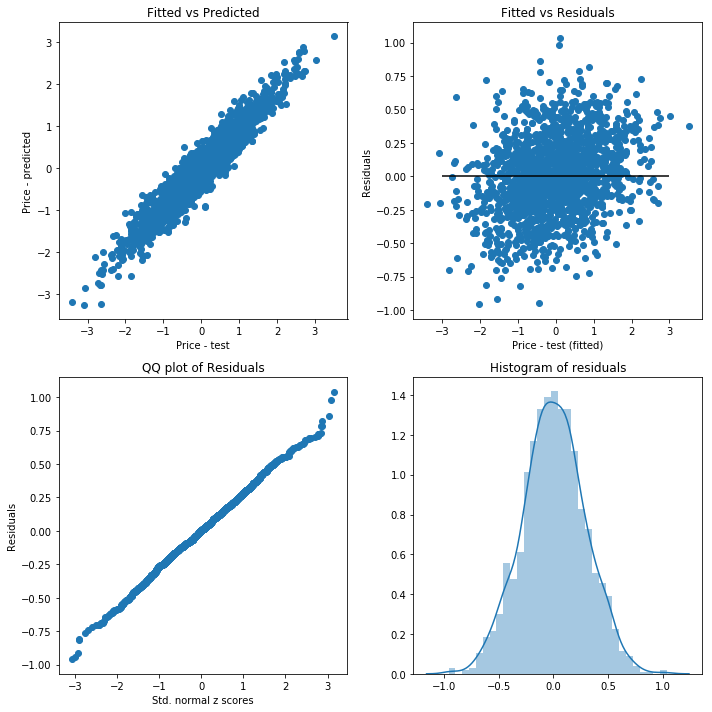From the charts above,

• Fitted vs predicted chart shows a strong correlation between the predictions and actual values
• Fitted vs Residuals chart shows an even distribution around the 0 mean line. There are not patterns evident, which means our model does not leak any systematic phenomena into the residuals (errors)
• Quantile-Quantile plot of residuals vs std. normal and the histogram of residual plots show a sufficiently normal distribution of residuals.

Thus all assumptions hold good.

#### Inverse Transform the scaled data and calculate RMSE¶¶

In :
Xscaled_train.columns

Out:
Index(['const', 'Avg Income', 'Avg House Age', 'Avg. Number of Rooms',
'Avg. Number of Bedrooms', 'Area Population'],
dtype='object')
In :
usa_house_fitted = Xscaled_test[Xscaled_test.columns[0:]]
usa_house_fitted['Price'] = yscaled_test

Out:
Avg Income Avg House Age Avg. Number of Rooms Avg. Number of Bedrooms Area Population Price
116 -0.572234 0.523958 0.333009 0.930840 -0.935132 -0.807544
1766 0.235111 -1.461858 -0.639184 -0.787131 -0.746915 -1.139124
318 -0.401314 -1.180810 0.886801 0.242031 -0.544216 -0.423747
1376 0.190042 -1.552636 0.291719 -0.503503 -1.186530 -1.552110
4699 -2.186060 -0.389114 -0.437924 -1.362489 0.903237 -1.453495
In :
usa_house_fitted_inv = s_scaler.inverse_transform(usa_house_fitted)
usa_house_fitted_inv = pd.DataFrame(usa_house_fitted_inv,
columns=usa_house_fitted.columns)

Out:
Avg Income Avg House Age Avg. Number of Rooms Avg. Number of Bedrooms Area Population Price
0 62484.855 6.497 7.323 5.13 26882.652 946943.036
1 71088.669 4.528 6.345 3.01 28750.641 829868.230
2 64306.339 4.807 7.880 4.28 30762.360 1082455.018
3 70608.372 4.438 7.281 3.36 24387.608 684049.919
4 45286.426 5.591 6.547 2.30 45127.832 718869.401
In :
yinv_predicted = (yscaled_predicted * s_scaler.scale_[-1]) + s_scaler.mean_[-1]

In :
yinv_predicted.shape

Out:
(1650, 1)
In :
usa_house_fitted_inv['Price predicted'] = yinv_predicted

Out:
Avg Income Avg House Age Avg. Number of Rooms Avg. Number of Bedrooms Area Population Price Price predicted
0 62484.855 6.497 7.323 5.13 26882.652 946943.036 1087455.848
1 71088.669 4.528 6.345 3.01 28750.641 829868.230 855848.404
2 64306.339 4.807 7.880 4.28 30762.360 1082455.018 971840.851
3 70608.372 4.438 7.281 3.36 24387.608 684049.919 877566.569
4 45286.426 5.591 6.547 2.30 45127.832 718869.401 743023.886

#### Calculate RMSE¶¶

RMSE root mean squared error. This is useful as it tell you in terms of the dependent variable, what the mean error in prediction is.

In :
mse_scaled = metrics.mean_squared_error(usa_house_fitted_inv['Price'],
usa_house_fitted_inv['Price predicted'])
numpy.sqrt(mse_scaled)

Out:
101796.27442079457
In :
mae_scaled = metrics.mean_absolute_error(usa_house_fitted_inv['Price'],
usa_house_fitted_inv['Price predicted'])
mae_scaled

Out:
80765.04773907141

### Conclusion¶¶

In this sample we observed two methods of predicting housing prices. The first involved applying linear regression on the dataset directly. The second involved scaling the features to standard normal distribution and applying a linear model using both sklearn and statsmodels packages. We thoroughly inspected the model parameters, vetted that assumptions hold good.

In the end, the accuracy of the models did not increase much after scaling. However, we were able to better determine which predictor variables were influential in a truest sense, not being biased by the scale of the units.

The allure of linear models is the explainability. They may not be the best when it comes to accurate predictions, however they help us answer basic questions better, such as “which characteristics influence the cost of my home, is it # of bedrooms or average income of the residents”?. The answer is the latter in this example.